# Curve

A curve is a shape or a line which is smoothly drawn in a plane having a bent or turns in it. For example, a circle is an example of curved-shape. In Mathematics, Geometry is a branch that deals with shapes, sizes, and the properties of figures. Geometry can be classified into two types. They are:

• Plane or Two Dimensional Geometry
• Solid or Three Dimensional Geometry

Two-dimensional geometry is the study of flat shapes like curves, lines, polygons, etc. that can be drawn on a piece of paper. On the other hand, three-dimensional geometry is the study of three-dimensional objects such as cubes, cylinders, spheres, and so on. Here we will discuss a fundamental element of plane geometry – curve in detail.

## Curve Meaning

A curve is defined as a smoothly- flowing continuous line that has bent. It does not have any sharp turns. The way to identify the curve is that the line bends and changes its direction at least once.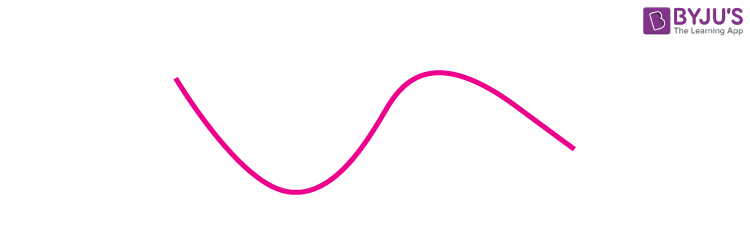### Curve Shape

Circles, ellipses, parabolas, and hyperbolas, as well as arcs, sectors and segments, are two-dimensional curved shapes. Whereas, shapes such as spheres, cylinders and cones, are referred to as three-dimensional curved shapes.

## Types of Curves

Curves are classified into different categories. Let us have a look at different types of curves with examples.

### Simple Curve

A curve that changes its direction, but it does not intersect itself. This type of curve is known as a simple curve. A simple curve may be open or closed.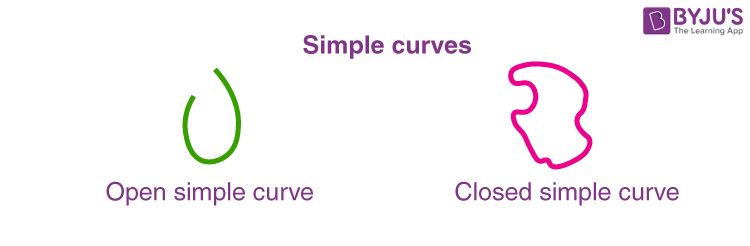### Non-Simple Curve

The non-simple curve is a type of curve that crosses its path. It means the curve intersects itself while changing its direction.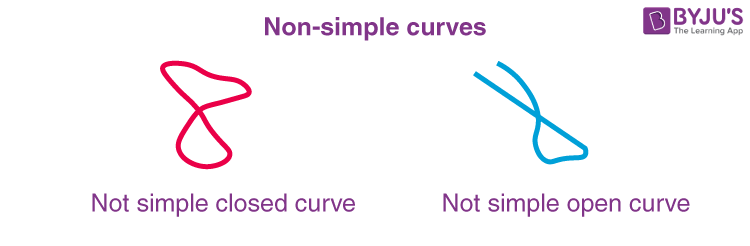### Open Curve

A curve has two endpoints, and when it does not enclose the area within itself it is known as an open curve. Some of the examples of open curves are as follows.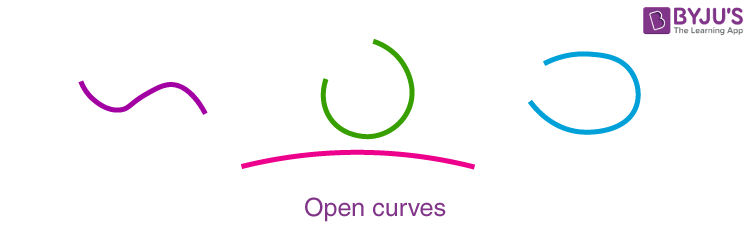### Closed Curve

A curve has no endpoints, and when it encloses the region or area will form, it is known as the closed curve. The type of curve is formed by joining the two endpoints of the open curve. The best example of closed curves are circles, ellipses, etc.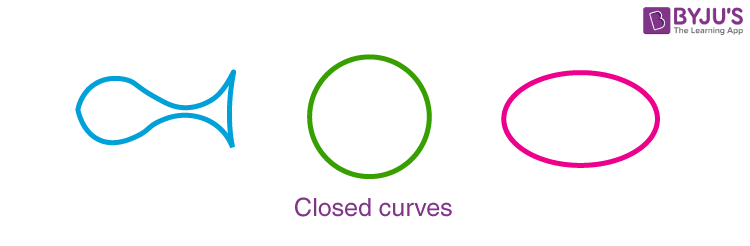### Upward Curve

A curve that points towards the upward direction is called an upward curve. The upward curves are called concave upward or convex downward curves.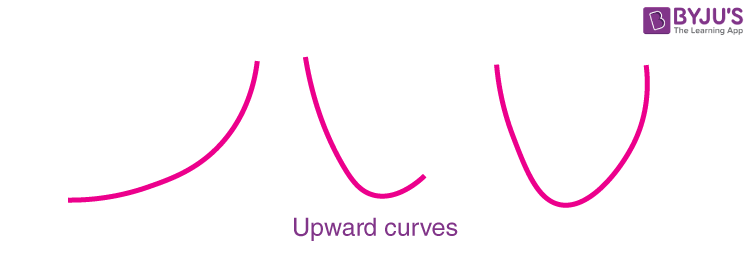### Downward Curve

A curve that points towards the downward direction is called a downward curve. The downward curves are called concave downward or convex upward curves.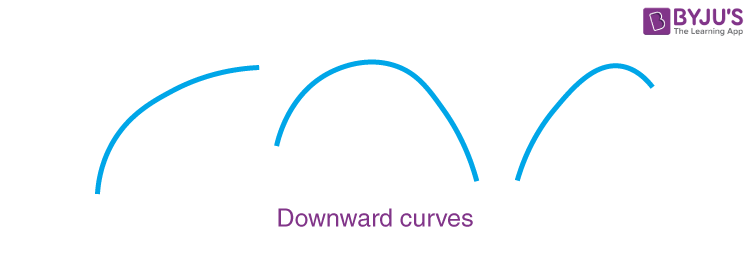### Curved Line

A curved line is a line that is not straight and is bent. If the curvature is not zero, then it is considered as a curve line. Generally, it is smooth and continuous.

### Curved Line Images

We can observe many objects and things which are in the shape of a curved line. Some of them include railway track at the turning points, track of a roller coaster, paths of a road particularly for hill areas and so on. Apart from the real life examples, we can also observe the curve shaped lines in maths such as graph a quadratic polynomial, i.e. parabola, ogive curve, arrows, etc. Below are the images of curve shaped lines.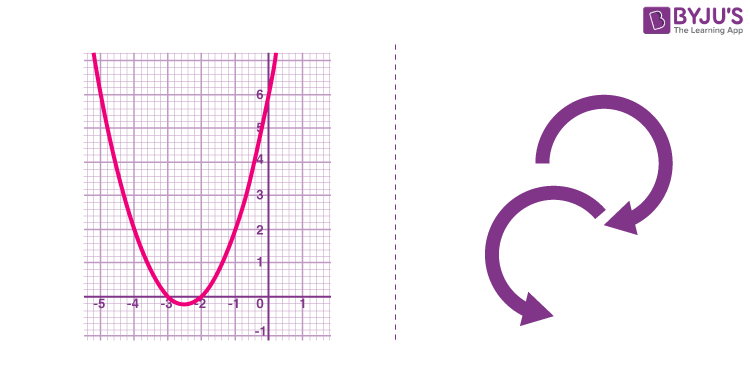### Area Between the curves

Area is a quantity that expresses the region covered for the given interval in the two-dimensional surface or a planar lamina. To find the area between the given two curves, it uses the concept of integration. The area between the two curves can be determined by calculating the difference between the integrals of two functions.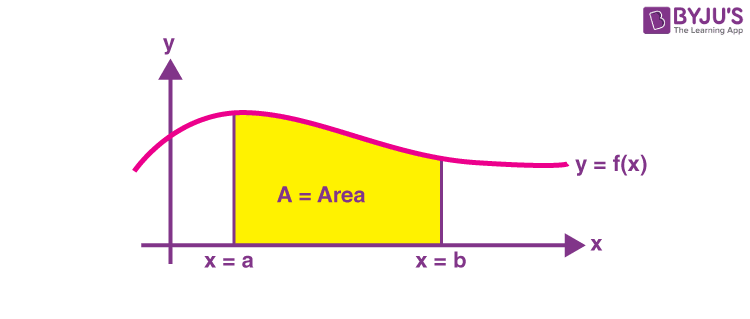The area between two curves that is measured between two values “a” and “b” is given by the integral from a to b of the given function. Here, the value of b is defined as the larger value than the value of a.

Therefore, the area between the two curves is defined as

$$\begin{array}{l}A = \int_{a}^{b}(f(x)-g(x))dx\end{array}$$

Where

f(x) is the curve with greater value.